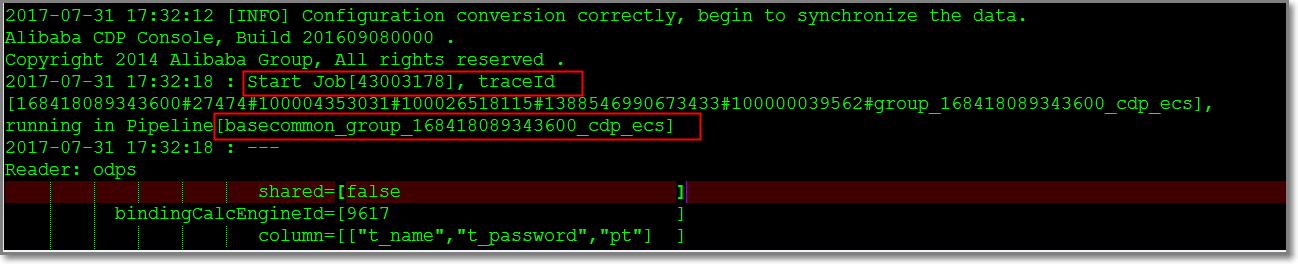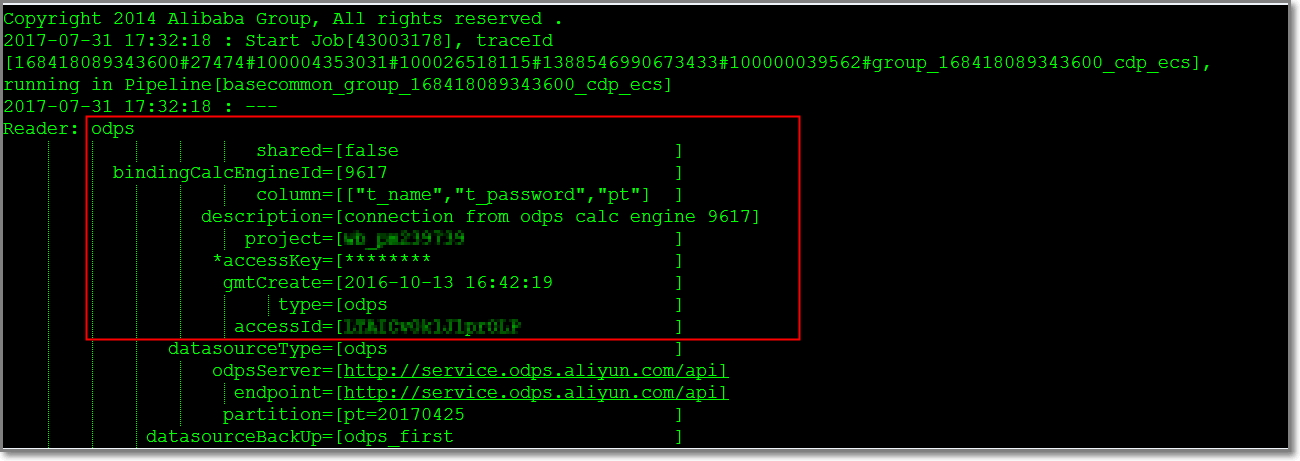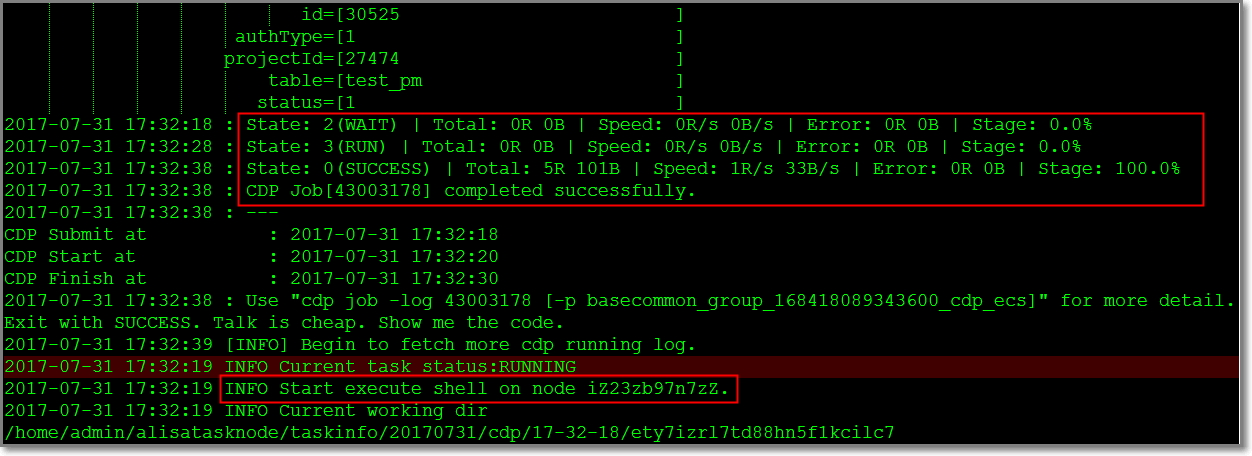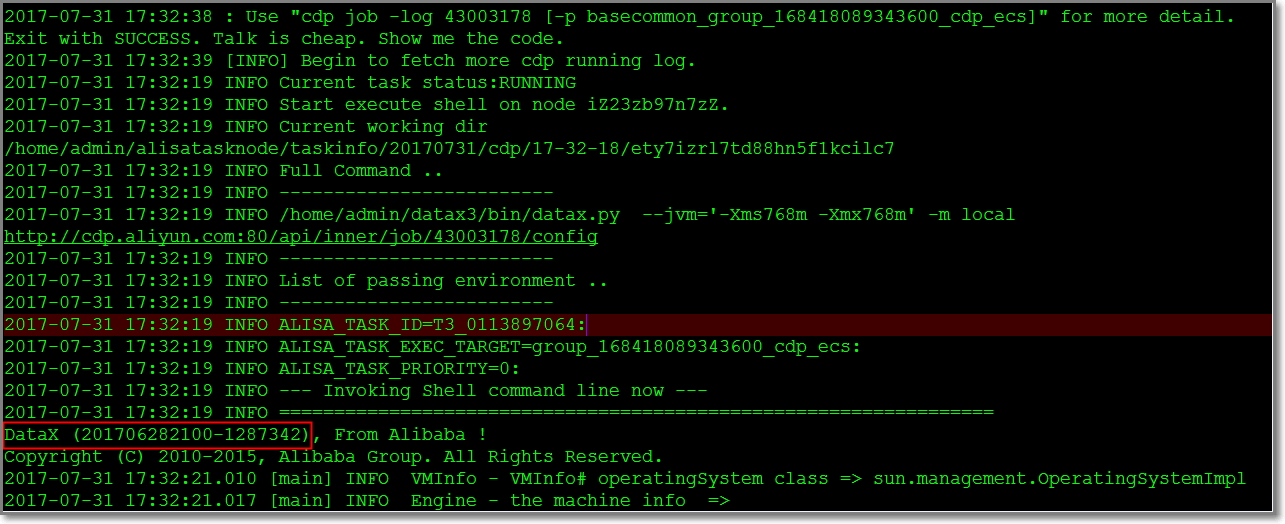All Products
Search
Document Center

# Sync log check

Last Updated: Apr 11, 2018

### Overview

Data Integration is a stable, efficient, and auto scalable data synchronization platform provided by the Alibaba Group. It is designed to implement fast and stable data movement and synchronization between various heterogeneous data sources in complex network environments. A wide range of data sources: text storage (FTP/SFTP/OSS/multimedia files), databases (RDS/DRDS/MySQL/PostgreSQL), NoSQL (Memcache/Redis/MongoDB/HBase), big data (MaxCompute/AnalyticDB/HDFS), and MPP database (HybridDB for MySQL).

As Data Integration enables interconnection between different types of databases in complex network environments, errors may occur while you use it. Next, let us analyze the log composition of Data Integration.

### Where does a task startAs shown in the preceding screenshot: Start Job means starting the job. The log under Start Job > running in Pipeline[XXXXX]“ is mainly used to identify the server on which the task is running. If XXXXX contains basecommon_group_XXXX, it means that the task is running on a server of a public resource group. If not, it runs on a server of your custom resource group. To view the name of the server running a task, see the description in section Task running status.

### Actual task running code

After a task starts, the log displays the actual task running code (for security reasons, sensitive information is indicated with an asterix (*)) as shown in the following figure.The actual code sample for the task in the figure is as follows.

``Reader: odps ``                       shared=[false                         ]``          bindingCalcEngineId=[9617                          ]``                       column=[["t_name","t_password","pt"]  ]``                  description=[connection from odps calc engine 9617]``                      project=[XXXXXXXXX                     ]``                   *accessKey=[********                      ]``                    gmtCreate=[2016-10-13 16:42:19           ]``                         type=[odps                          ]``                     accessId=[XXXXXXXXX                     ]``               datasourceType=[odps                          ]``                   odpsServer=[http://service.xxx.aliyun.com/api]``                     endpoint=[http://service.xxx.aliyun.com/api]``                    partition=[pt=20170425                   ]``             datasourceBackUp=[odps_first                    ]``                         name=[odps_first                    ]``                     tenantId=[168418089343600               ]``                      subType=[                              ]``                           id=[30525                         ]``                     authType=[1                             ]``                    projectId=[27474                         ]``                        table=[t_name                        ]``                       status=[1                             ]``Writer: odps ``                       shared=[false                         ]``          bindingCalcEngineId=[9617                          ]``                       column=[["id","name","pt"]            ]``                  description=[connection from odps calc engine 9617]``                      project=[XXXXXXXXX                     ]``                   *accessKey=[********                      ]``                    gmtCreate=[2016-10-13 16:42:19           ]``                         type=[odps                          ]``                     accessId=[XXXXXXXXX                     ]``               datasourceType=[odps                          ]``                   odpsServer=[http://service.xxx.aliyun.com/api]``                     endpoint=[http://service.xxx.aliyun.com/api]``                    partition=[                              ]``                     truncate=[true                          ]``             datasourceBackUp=[odps_first                    ]``                         name=[odps_first                    ]``                     tenantId=[XXXXXXXXX                     ]``                      subType=[                              ]``                           id=[30525                         ]``                     authType=[1                             ]``                    projectId=[27474                         ]``                        table=[test_pm                       ]``                       status=[1                             ]``

This is a typical task code used to synchronize a MaxCompute (formerly known as ODPS) data source to a MaxCompute data source. For analysis on the task code, see MaxCompute Reader and MaxCompute Writer.

### Task running status

We have introduced the actual task running code, which is followed by the running status of the task, as shown in the following figure.The content included in the larger red box records the start and end time of the task.

• When Status: 2(WAIT) is displayed, it means that the task is waiting.

• When Status: 3(RUN) is displayed, it means that the task is running.

• When Status: 0(SUCCESS) is displayed, it means that the task is completed successfully.

Note: Under the record indicating task completion is INFO Start run shell on node XXXXXXX, which means that the task actually runs on XXXXXXX server.

Troubleshooting assistant: Dirty data cannot be written in, and the log reports the following error in case of dirty data

### Detailed running logs

In fact, the task log of data synchronization has ended already. The following log is the detailed running log of DataX (Data Integration applies encapsulation to the open-source project DataX of Alibaba), as shown in the following figure.If the problem still persists even after referring to the common errors article, contact us.